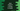# Java program to check if a string is empty or not## Java program to check if a string is empty or not:

In this post, we will learn how to check if a string is empty or not in Java. Java string provides a method called isEmpty() that checks if a string is empty or not. In this post, we will learn the definition of isEmpty() and how to use it with examples.

### Definition of isEmpty():

The isEmpty() method is defined as like below:

``str.isEmpty()``

Where, str is the string object we are calling isEmpty on.

### Parameter and return values:

The isEmpty method doesn’t take any parameter. It returns a boolean value, true if the string is empty, else false.

### How to check if a string is empty string or not:

We can use the isEmpty() method to check if a string is empty or not. Let’s try it with an example:

``````class Main {
public static void main(String[] args) {
String[] arr = {"hello", "", "   ", "#", "."};

for (String s : arr) {
System.out.println(s + ":" + s.isEmpty());
}
}
}``````

If you run this program, it will print the below output:

``````hello:false
:true
:false
#:false
.:false``````

As you can see here, it returns false for strings with multiple blank spaces. If we check the definition of isEmpty, it is defined as like below:

``````public boolean isEmpty() {
return value.length == 0;
}``````

It checks the length of the string, if the string length is 0, it returns true.

### isEmpty() with null string:

It throws NullPointerException if you use isEmpty() with a null string. For example,

``````class Main {
public static void main(String[] args) {
String givenString = null;
System.out.println(givenString.isEmpty());
}
}``````

It will throw NullPointerException:

``````Exception in thread "main" java.lang.NullPointerException
at Main.main(Main.java:4)``````

### isEmpty() with a string that is not initialized:

If we try to use isEmpty() on a string that is not initialized, it will show an error that variable string might not have been initialized.

### How to check if a string is null or empty:

If you want to check if a string is null or empty, we have to combine multiple checks. We can add checks:

• to check if a string is null or not
• to check if a string is empty or not

We can also trim a string before we check it to handle strings with multiple blank spaces.

For example:

``````class Main {
private static boolean isNullOrEmpty(String str) {
return str == null || str.trim().isEmpty();
}

public static void main(String[] args) {
String[] arr = {"", "  ", "\t", "\n", "hello", null};

for (String str : arr) {
System.out.println(str + ":" + isNullOrEmpty(str));
}
}
}``````

We created a new method isNullOrEmpty that returns true if the parameter string is either null or empty. We are checking if the string is null or if the trimmed value of the string is empty.

If you run the above program, it will print the output as like below:

``````:true
:true
:true

:true
hello:false
null:true``````